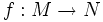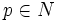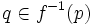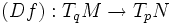# Critical value

Let$f:M \to N$ be a smooth map between differential manifolds$M$ and$N$. A point$p \in N$ is termed a critical value for$f$ if it satisfies the following condition:
• There exists$q \in f^{-1}(p)$ such that$q$ is a critical point; in other words, the map$(Df): T_qM \to T_pN$ is not surjective. In other words, it is the image of a critical point.
• It is not a regular value of$f$.$N$ is thus partitioned into two subsets: the critical values of$f$, and the regular values of$f$.